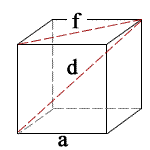## Cube Calculator

 a = Units: S = 0 V = 0 f = 0 d = 0

a = side lengths, f = face diagonal, d = solid diagonal, S = surface area, V = volume

» Show Work

A cube is a 3D figure that has six square faces, with three meeting at each vertex. For more information on calculating properties of a cube, please read the in-depth explanation section below.

To calculate the side lengths, solid diagonal, face diagonal, surface area, and volume of a cube, you need only one of these values. If you have one of these five measurements, use the subsection below depending on which measurement you have.### Cube Formulas

Cube Surface Area = 6a2

For example, when a = 3

1. To calculate S, use the following formula: 6a2. When you substitute a for its value, you get 6(32). First square a because exponents have a higher precedence than multiplication. 32 = 9. The formula now looks like this: 6(9), which equals 54 m2.
2. To calculate V, use the following formula: a3. When you substitute a for its value, you get 33, which equals 27 m3.
3. To calculate f, use the following formula: a√2. When you substitute a for its value, you get 3√2, which equals 4.24264 m.
4. To calculate d, use the following formula: a√3. When you substitute a for its value, you get 3√3, which equals 5.19615 m.

Thinkcalculator.com provides you helpful and handy calculator resources.